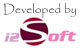Monday, 29 May, 2023, Time : 4:03 PM

• ক = k
• খ = kh, K
• গ = g
• ঘ = gh,G
• ঙ = Ng
• চ = c
• ছ = ch, C
• জ = j
• ঝ = jh, J
• ঞ = NG
• ট = t
• ঠ = th
• ড = d
• ঢ = dh
• ণ = N
• ত = T
• থ = Th
• দ = D
• ধ = Dh
• ন = n
• প = p
• ফ = ph, f
• ব = b
• ভ = bh, v
• ম = m
• য = z
• র = r
• ল = l
• শ = sh
• ষ = S
• স = s
• হ = h
• ক্ষ = k+S
• ড় = R
• ঢ় = Rh
• য় = y
• ৎ = tt
• ং = ng
• ঃ = H
• ঁ = NN
• অ = Ao
• আ = a, A
• ই = i
• ঈ = II
• উ = U
• ঊ = UU
• ঋ = WR
• এ = E
• ঐ = OI
• ও = O
• ঔ = OU

• k+l = ক্ল
• k+l = ক্ল
• s+t = স্ট
• S+N = ষ্ণ
• T+T+b = ত্ত্ব
• Ng+k = ঙ্ক
• Ng+g = ঙ্গ
• j+NG = জ্ঞ
• NG+c = ঞ্চ
• NG+j = ঞ্জ
• NG+C = ঞ্ছ
• N+d = ণ্ড
• ব ফলা = +b
• য ফলা = Y
• র ফলা = +r
• রেফ = r+
• ঋ কার = wr
• ্(হসন্ত) = HH, +
• ডট(.) = ..
• ে = e
• ৈ = oi
• ো = o
• ৌ = ou
• ু = u
• ূ = uu
• ি = i
• ী = ii
• া = a
• ।(দাড়ি) = .